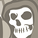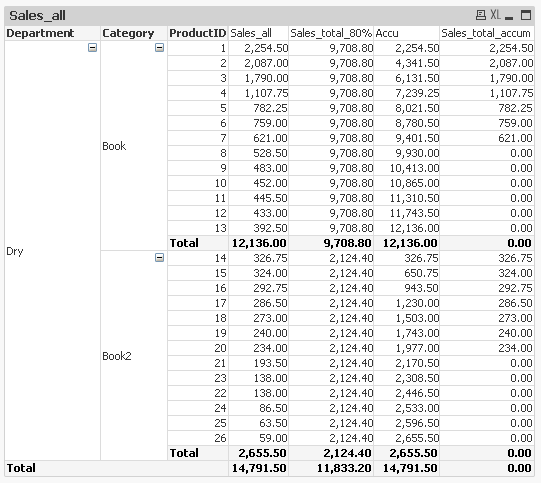# QlikView App Dev

Discussion Board for collaboration related to QlikView App Development.

Announcements
LINKEDIN LIVE: Democratizing data to enhance customer-centricity. JULY 29TH REGISTER TODAY
cancel
Showing results for
Did you mean:Contributor II

## Need help with Expression (Accummulate and sum)

I do Accumulate report by Pivot Chart like this;I'm stuck with 'Sales_total_accum' => I do in If Clause; If(Accu<=Sales_total_80%,Sum(Sales_All),0).

But no number in 'Total' level, I would like to sum it up. So I need you suggestion and help .You can view my solution in attach.

Thanks.

Labels (7)

• ### Set Analysis

1 Solution

Accepted SolutionsMVP

Try this

`=Sum(Aggr(If(RangeSum(Above(Sum(Sales_All), 0, RowNo())) <= Sum(TOTAL <Department, Category> Sales_All)*0.8, Sum(Sales_All), 0), Department, Category, ProductID))`

or this if you have QV12

`=Sum(Aggr(If(RangeSum(Above(Sum(Sales_All), 0, RowNo())) <= Sum(TOTAL <Department, Category> Sales_All)*0.8, Sum(Sales_All), 0), Department, Category, (ProductID, (NUMERIC))))`
2 RepliesMVP

Try this

`=Sum(Aggr(If(RangeSum(Above(Sum(Sales_All), 0, RowNo())) <= Sum(TOTAL <Department, Category> Sales_All)*0.8, Sum(Sales_All), 0), Department, Category, ProductID))`

or this if you have QV12

`=Sum(Aggr(If(RangeSum(Above(Sum(Sales_All), 0, RowNo())) <= Sum(TOTAL <Department, Category> Sales_All)*0.8, Sum(Sales_All), 0), Department, Category, (ProductID, (NUMERIC))))`Contributor II
Author
It's working very fine, Thank you so much 🙂Community Browser